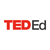# The last banana: A thought experiment in probability - Leonardo Barichello

• 1,492,170 Views
• TEDEd Animation

#### Let’s Begin…

Imagine a game played with two players and two dice: if the biggest number rolled is one, two, three, or four, player 1 wins. If the biggest number rolled is five or six, player 2 wins. Who has the best probability of winning the game? Leonardo Barichello explains how probability holds the answer to this seemingly counterintuitive puzzle.

373

Create and share a new lesson based on this one.

TED-Ed Animations feature the words and ideas of educators brought to life by professional animators. Are you an educator or animator interested in creating a TED-Ed Animation? Nominate yourself here »

## Meet The Creators

• Educator Leo Barichello
• Director Richard O'Connor
• Animator Taisiya Zaretskaya, Marisha Falkovich
• Script Editor Eleanor Nelsen
Additional Resources for you to Explore
Examples using dice and coins are common in any probability or statistic lesson, not only because they are simple, but because they are also very powerful in describing a huge variety of important experiments. Find out more about probabilistic experiments like the rolling of two dice here. For more probabilities fun, watch This Is Mathematics Episode 8. Where can you apply probability in your everyday life?

Probability can be applied to real-life situations. Medical decisions, extreme weather events and batting averages are just three examples. The Math Forum at Drexel and NRICH: Enriching Mathematics provide more ideas about this topic. Take a chance and check them out.

Let's talk about the TED-Ed Lesson with the two castaways playing dice for the last banana. As you saw, there were two different strategies to calculate the theoretical probability of each player winning the proposed game.

The first strategy, based on the list obtained through a table, involved all equiprobable events. If one can build such list, it is easy to calculate the probability of any set of possible outcomes by dividing the number of relevant outcomes by the total of events. Using a table to list all equiprobable events is useful when the experiment can be broken down into two basic actions such as rolling two dice or rolling one die and flipping one coin. This probability game called Dice Race simulates different scenarios involving two dice. Which horse do you think will win? Is there any way to increase your chance of predicting the outcome?

The second strategy shown in the lesson was to calculate using the joint probability of independent events. Although this approach seems to be simple (just multiply fractions), it relies on more formal knowledge about probability. If you are interested, seek more answers here. They combine theory with some games like the one shown in the lesson.

What are spinners and urns? Two models commonly used in probability. Both are more flexible than dice and coins, because you can vary their structure in order to generate more complex situations. This online interactive spinner can help you design your own experiment on probability.

Finally, if you want to dig a little deeper in the terrain of probability, this video from Numberphile: 1,296 and Yahtzee can be very intriguing! Looking for something to do? Play Yahtzee and see how long it takes you to roll 5 dice with all the same value!TED-Ed
Lesson Creator
New York, NY
02/23/2015 •
51 Responses

Create and share a new lesson based on this one.